• 12
•
•
•
•
•
•
•
12
Shares

# ELECTRIC DIPOLE IN UNIFORM EXTERNAL ELECTRIC FIELD

In the previous article of physics, we have talked about the concept of electric charges, electric field, electric dipole and dipole moment in detail. If you think that you need to brush up your concepts again, then you can go for the same just by clicking above link.

In this article we are going to derive the expression for the torque experienced by an electric dipole in the uniform external electric field. So let’s get started…

Imagine of a small electric dipole with +q and -q electric charges and which is seperated by the distance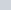is kept in the uniform external electric field. Keep in mind that the electric dipole which you have imagined is making anglewith the electric field. As you can see below.When we put a electric dipole in uniform external electric field with anglethen the charges of the dipole experiences a electric force. The experienced electric force is given as-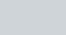The expression which are you seeing in equ(1) is the force experienced by the charges of the dipole, when it is kept in uniform external electric field. The above force is equal in magnitude but opposite in direction. But the interesting thing which occurred with the electric dipole after putting it into the external electric field is that it start showing coupling effect.

The coupling effect constitutes, when two forces are equal in magnitude and opposite in direction and acts in different points. This coupling effect leads dipole to rotation with centred O and it is termed as torque. Here points to be remembered is that Whenever a torque present there exists a rotation.

(1)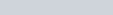The net torque τ acts on the dipole about an axis passing through the midpoint O of the dipole. The torque on the dipole is given as the product of the either force and the perpendicular distance between the parallel forces.
Mathematically it is given as –Put the value of BN in above equation.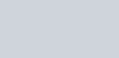In the vector formis given as –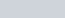The SI unit of torque is Newton-metre (N-m) and it’s dimensional formula is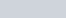### SPECIAL CASES

1). If θ = 0°, then τ = 0

If this situation occurres then the dipole is in stable condition with no rotation. In this situation dipole is totally aligned with the electric field.

2). If θ = 90°, then τ = pE (maximum value)

If If this situation occurres then the torque on the dipole is at maximum value. In this situation dipole is perpendicular to the electric field.

3). If θ = 180°, then τ = 0

If this situation occurres then the dipole is in unstable condition. Because the orientation of the charges changes. The positive charge goes on left side and the negative charge goes on right side, this makes dipole unstable. This orientation alter the equilibrium of the dipole.